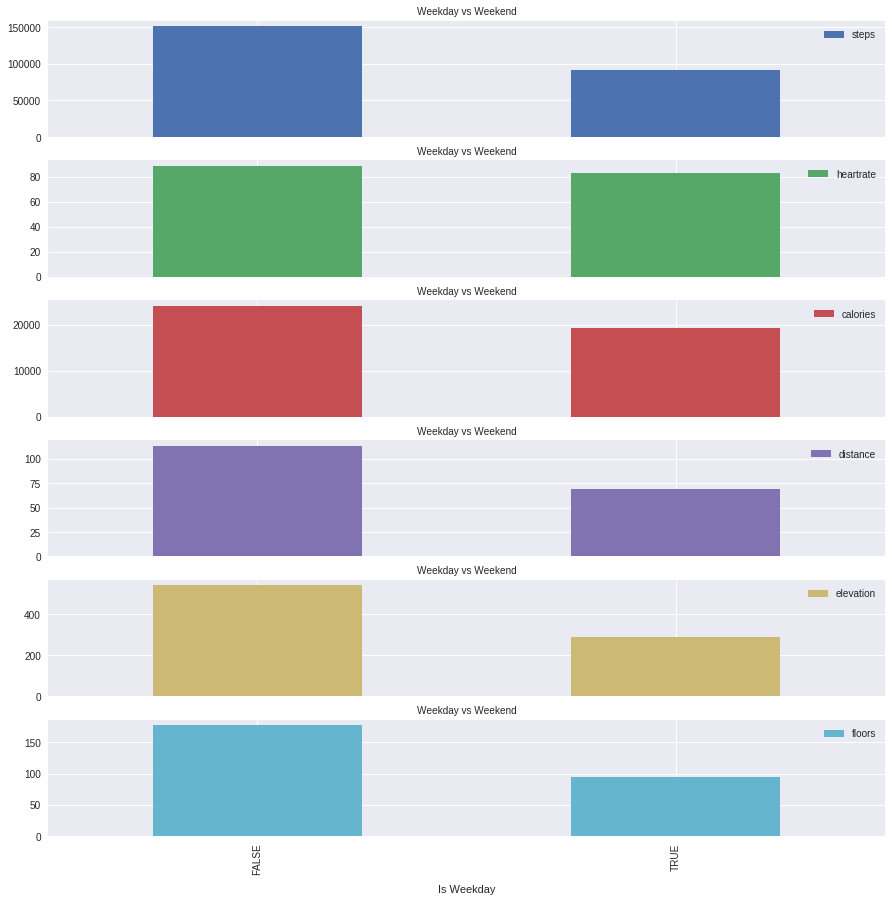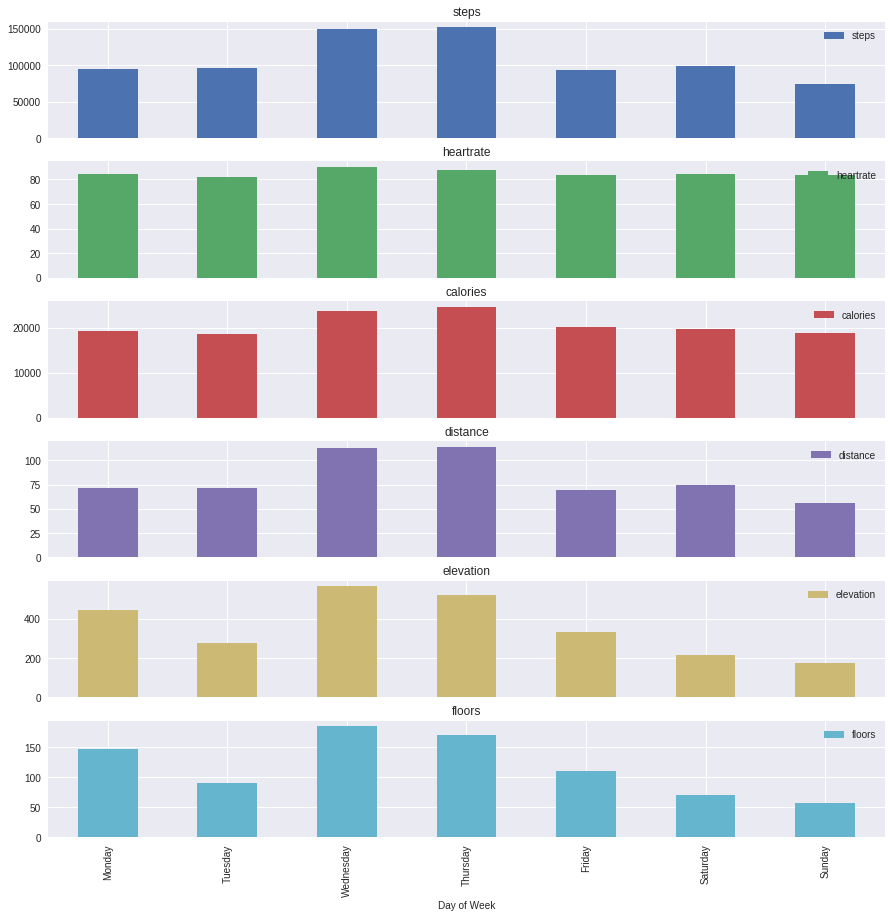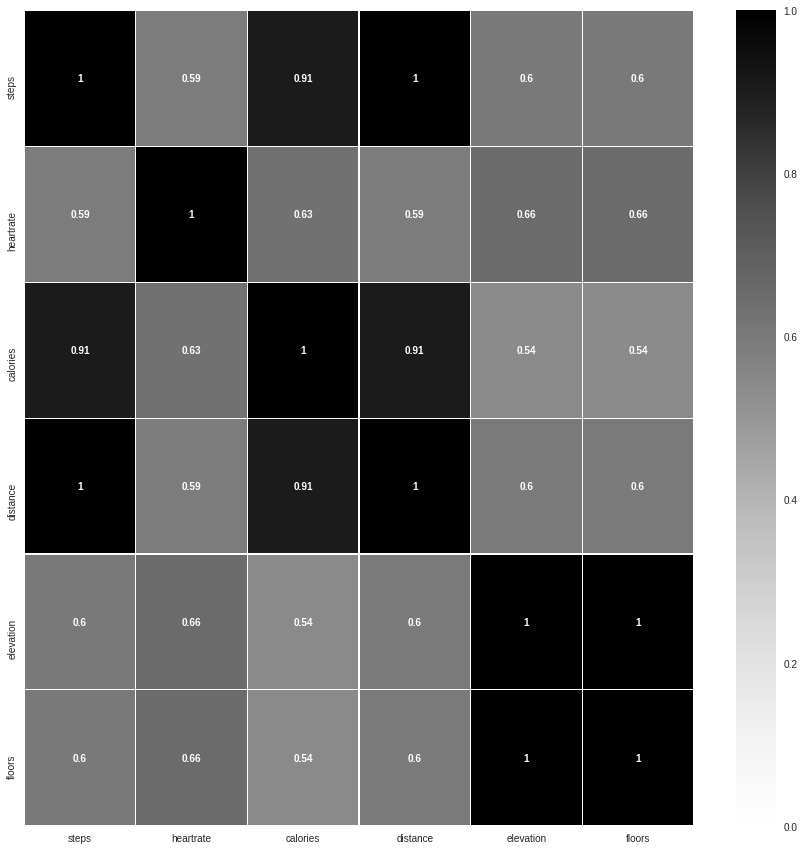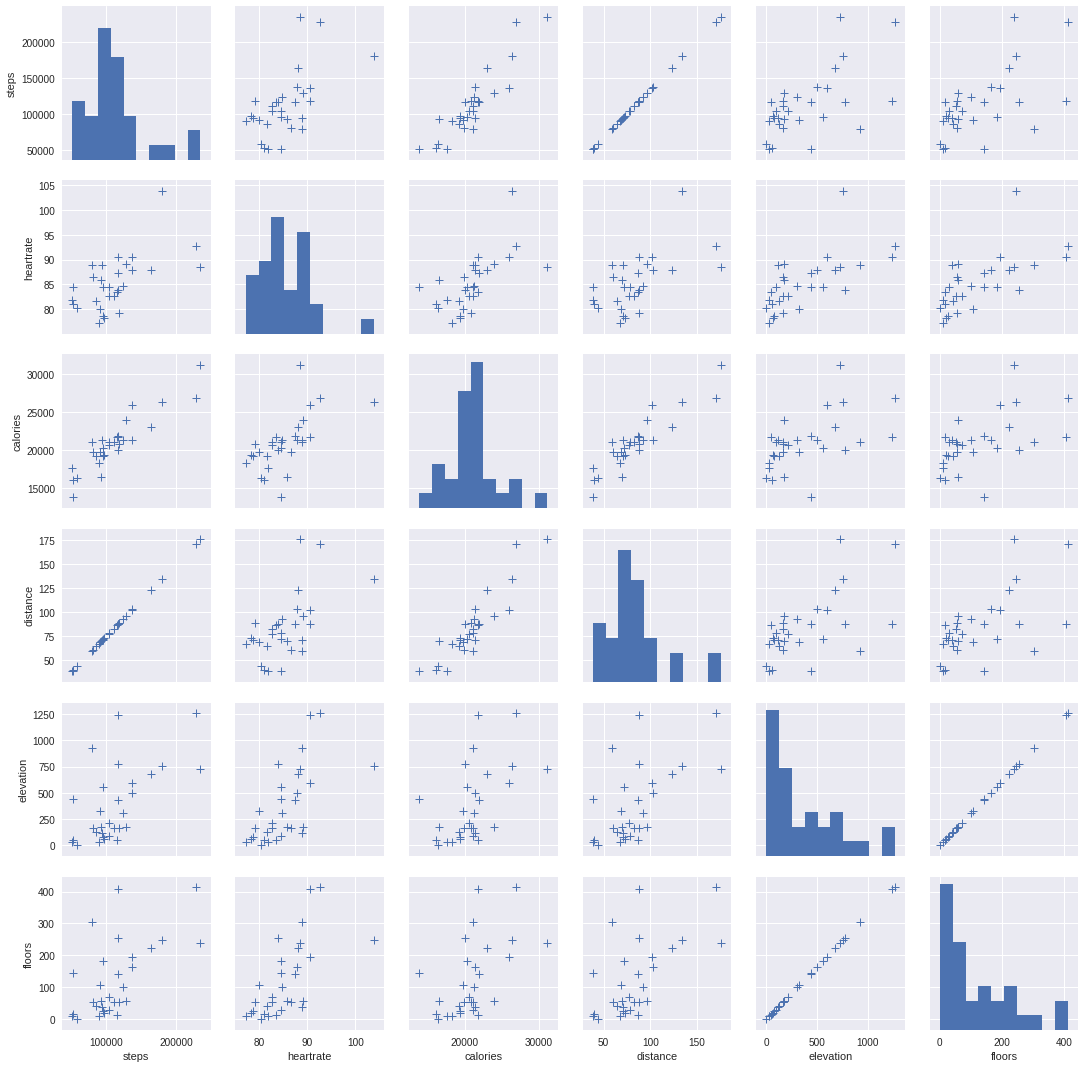# Details for `fitbit_intraday_analysis.ipynb`

#### Description

Fitbit intraday data analysis

#### Tags & Data Sources

fitbit intraday Fitbit Intraday

#### Comments

Please log in to comment.

### Notebook Last updated 3 weeks, 2 days ago

This notebook extract the weight log from fitbit Data. We will analyze the change of weight overtime.

First, let's try to load necessary packages and import your data.

In :
```import pandas as pd
import json
import numpy as np
import matplotlib.pyplot as plt
import seaborn as sns
import urllib
import requests
import os
import tempfile
from datetime import datetime

master_token = 'WEvvgv0KUJh8CxbxxYwzAU24SFWMJcdwwjUzmtt9jr2w0wBkDoLftKyyLl60KPD7'
response = requests.get('https://www.openhumans.org/api/direct-sharing/project/exchange-member/'
'?access_token={}&project_member_id=36032055'.format(master_token))
Fitbit_intraday = [x for x in response.json()['data'] if x['source'] == 'direct-sharing-191']
```

Let's extract the weight log of certain month from the data. You can change the date if you want.

In :
```month_of_interest = '2019-06'
```

Let's get your activities intraday data and convert into a dataframe for further analysis.

In :
```steps = []
time = []
for i in Fitbit_intraday:
if i['basename'] == 'fitbit-intraday-{}.json'.format(month_of_interest):
Month_fitbit_intraday = json.loads(requests.get(i['download_url']).content)
for day in Month_fitbit_intraday['activities-steps-intraday']:
for dpoint in day['dataset']:
steps.append(dpoint['value'])
time.append(datetime.strptime(day['date'] +' '+ dpoint['time'][:6]+'00','%Y-%m-%d %H:%M:%S'))

df_step = pd.DataFrame(
data = {
'DateTime': time,
'steps': steps,
}
)

df_step = df_step.set_index('DateTime', drop= False)
df_step['Date'] = df_step.index.date
df_step['Time'] = df_step.index.time
df_step['DayofWeek'] = df_step.index.weekday_name
```
In :
```heartrate = []
time = []

for day in Month_fitbit_intraday['activities-heart-intraday']:
for dpoint in day['dataset']:
heartrate.append(dpoint['value'])
time.append(datetime.strptime(day['date'] +' '+ dpoint['time'][:6]+'00','%Y-%m-%d %H:%M:%S'))

df_heartrate= pd.DataFrame(
data = {
'DateTime': time,
'heartrate': heartrate,

}
)
```
In :
```calories = []
time = []

for day in Month_fitbit_intraday['activities-calories-intraday']:
for dpoint in day['dataset']:
calories.append(dpoint['value'])
time.append(datetime.strptime(day['date'] +' '+ dpoint['time'][:6]+'00','%Y-%m-%d %H:%M:%S'))

df_calories= pd.DataFrame(
data = {
'DateTime': time,
'calories': calories,

}
)
```
In :
```distance = []
time = []

for day in Month_fitbit_intraday['activities-distance-intraday']:
for dpoint in day['dataset']:
distance.append(dpoint['value'])
time.append(datetime.strptime(day['date'] +' '+ dpoint['time'][:6]+'00','%Y-%m-%d %H:%M:%S'))

df_distance= pd.DataFrame(
data = {
'DateTime': time,
'distance': distance,

}
)
```
In :
```elevation = []
time = []

for day in Month_fitbit_intraday['activities-elevation-intraday']:
for dpoint in day['dataset']:
elevation.append(dpoint['value'])
time.append(datetime.strptime(day['date'] +' '+ dpoint['time'][:6]+'00','%Y-%m-%d %H:%M:%S'))

df_elevation= pd.DataFrame(
data = {
'DateTime': time,
'elevation': elevation,

}
)
```
In :
```floors = []
time = []

for day in Month_fitbit_intraday['activities-floors-intraday']:
for dpoint in day['dataset']:
floors.append(dpoint['value'])
time.append(datetime.strptime(day['date'] +' '+ dpoint['time'][:6]+'00','%Y-%m-%d %H:%M:%S'))

df_floors = pd.DataFrame(
data = {
'DateTime': time,
'floors': floors,

}
)
```
In :
```df_activities = pd.merge(df_step,df_heartrate, on='DateTime', how='outer' )
df_activities = pd.merge(df_activities,df_calories, on='DateTime', how='outer' )
df_activities = pd.merge(df_activities,df_distance, on='DateTime', how='outer' )
df_activities = pd.merge(df_activities,df_elevation, on='DateTime', how='outer' )
df_activities = pd.merge(df_activities,df_floors, on='DateTime', how='outer' )
df_activities =  df_activities.set_index('DateTime', drop= False)
df_activities.head(10)
```
```/opt/conda/lib/python3.6/site-packages/IPython/core/interactiveshell.py:2963: FutureWarning: 'DateTime' is both an index level and a column label.
Defaulting to column, but this will raise an ambiguity error in a future version
exec(code_obj, self.user_global_ns, self.user_ns)
```
Out:
DateTime steps Date Time DayofWeek heartrate calories distance elevation floors
DateTime
2019-06-01 00:00:00 2019-06-01 00:00:00 0 2019-06-01 00:00:00 Saturday 84.0 1.4313 0.0 0.0 0
2019-06-01 00:00:00 2019-06-01 00:00:00 0 2019-06-01 00:00:00 Saturday 86.0 1.4313 0.0 0.0 0
2019-06-01 00:00:00 2019-06-01 00:00:00 0 2019-06-01 00:00:00 Saturday 86.0 1.4313 0.0 0.0 0
2019-06-01 00:00:00 2019-06-01 00:00:00 0 2019-06-01 00:00:00 Saturday 85.0 1.4313 0.0 0.0 0
2019-06-01 00:00:00 2019-06-01 00:00:00 0 2019-06-01 00:00:00 Saturday 86.0 1.4313 0.0 0.0 0
2019-06-01 00:01:00 2019-06-01 00:01:00 0 2019-06-01 00:01:00 Saturday 87.0 1.4313 0.0 0.0 0
2019-06-01 00:01:00 2019-06-01 00:01:00 0 2019-06-01 00:01:00 Saturday 89.0 1.4313 0.0 0.0 0
2019-06-01 00:01:00 2019-06-01 00:01:00 0 2019-06-01 00:01:00 Saturday 90.0 1.4313 0.0 0.0 0
2019-06-01 00:01:00 2019-06-01 00:01:00 0 2019-06-01 00:01:00 Saturday 89.0 1.4313 0.0 0.0 0
2019-06-01 00:01:00 2019-06-01 00:01:00 0 2019-06-01 00:01:00 Saturday 87.0 1.4313 0.0 0.0 0

Let's convert your intraday data into daily summary first. For 'steps', 'calories','distance','elevation','floors', we use daily sum while for 'heartrate', we use daily average.

In :
```df_activities_daily = df_activities.resample('D').sum()
df_activities_daily = pd.DataFrame(df_activities_daily)
df_activities_daily['heartrate'] = df_activities['heartrate'].resample('D').mean()
df_activities_daily['DayofWeek'] = df_activities_daily.index.weekday_name

weekday = ['Monday','Tuesday','Wednesday','Thursday','Friday']
weekend = ['Saturday','Sunday']

df_activities_daily.loc[df_activities_daily['DayofWeek'].isin(weekday), 'IsWeekday'] = 'TRUE'
df_activities_daily.loc[df_activities_daily['DayofWeek'].isin(weekend), 'IsWeekday'] = 'FALSE'
```

Let's first take a look at if there is different between weekdays' data and weekends' data.

In :
```df_activities_daily.groupby('IsWeekday').mean()
```
Out:
steps heartrate calories distance elevation floors
IsWeekday
FALSE 151317.40 88.718781 24143.135279 113.364920 541.629617 177.7
TRUE 91778.55 83.482381 19315.971446 68.781705 289.560008 95.0
In :
```cols_plot = ['steps', 'heartrate', 'calories','distance','elevation','floors']
axes = df_activities_daily.groupby('IsWeekday')[cols_plot].mean().plot(kind='bar', figsize=(15, 15), subplots=True)
for ax in axes:
ax.set_xlabel('Is Weekday', labelpad=10)
ax.set_title('Weekday vs Weekend', fontsize=10)
plt.show()
```Then, let's explore the differences based on the day of a week.

In :
```dayAverage = df_activities_daily.groupby('DayofWeek').mean()
dayAverage
```
Out:
steps heartrate calories distance elevation floors
DayofWeek
Friday 95527.75 84.160237 19163.565539 71.541300 446.532013 146.50
Monday 96138.25 82.073093 18502.222291 72.066750 275.844007 90.50
Saturday 150238.20 90.036896 23645.868651 112.487900 564.489617 185.20
Sunday 152396.60 87.400667 24640.401906 114.241940 518.769617 170.20
Thursday 93033.25 83.304776 20250.968187 69.732775 334.518009 109.75
Tuesday 99352.00 84.018028 19772.280900 74.474475 215.646007 70.75
Wednesday 74841.50 83.855770 18890.820314 56.093225 175.260006 57.50
In :
```axes = df_activities_daily.groupby('DayofWeek')[cols_plot].mean().plot(kind='bar', figsize=(15, 15), subplots=True)
plt.xlabel('Day of Week', fontsize=10)
plt.xticks(np.arange(7), weekday + weekend, rotation=90, fontsize =10)
plt.show()
```We can also explore the correlations between these activity variables.

In :
```correlations = df_activities_daily[cols_plot].corr()
fig = plt.figure(figsize=(15,15))
sns.heatmap(correlations,cmap=plt.cm.Greys, linewidths=0.05,vmax=1, vmin=0 ,annot=True,annot_kws={'size':10,'weight':'bold'})
ax.set_title('Activities correlation')
plt.show()
```In :
```sns.pairplot(df_activities_daily[cols_plot].dropna(), kind="scatter", markers="+", plot_kws=dict(s=50, edgecolor="b", linewidth=1))
plt.show()
```In [ ]:
```
```